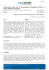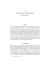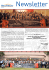# Kepler`s Laws, Binaries, and Stellar Masses

## Transcription

Kepler`s Laws, Binaries, and Stellar Masses
```Ay 20
Fall 2004
Kepler’s
Laws,
Binaries,
and Stellar
Masses
Kepler’s Laws:
1. The orbits of planets are
elliptical, with the Sun at a
focus
sweep out equal areas per
unit time
3. Squares of orbital periods
are proportional to cubes
of semimajor axes:
P 2 [yr] = a pl 3 [au]
• Derived empirically from Tycho de Brahe’s data
• Explained by the Newton’s theory of gravity
Orbits in a Gravitational Potential
For a point mass (or spherically symmetric one), they are
always the conic sections:
The shape depends on the sign of
the total energy, E tot = E kin - E pot :
E tot < 0 Ÿ Ellipse
E tot = 0 Ÿ Parabola
E tot > 0 Ÿ Hyperbola
For the elliptical orbits, the
eccentricity depends on the
angular momentum: circular
orbits have the maximum ang.
mom. for a given energy
Kepler’s 2nd Law:
A quick and simple derivation
Angular momentum, at any time: J = Mpl V r = const.
Thus: V r = const. (this is also an “adiabattic invariant”)
Element of area swept: dA = V r dt
Sectorial velocity: dA/dt = V r = const.
Independent of Mpl !
It is a consequence of
the conservation of
angular momentum.
r
V
Planets move slower at the
aphelion and faster at the perihelion
Kepler’s 3rd Law:
A quick and simple derivation
F cp = G Mpl M§ / (apl + a§) 2
≈ G Mpl M§ / apl 2
(since Mpl << M§, apl >> a§ )
F cf = Mpl Vpl2 / apl
= 4 p 2 Mpl apl / P 2
(since Vpl = 2 p apl / P )
F cp = F cf
Ÿ 4 p 2 apl 3 = G M§ P 2 (independent of Mpl !)
Another way: E kin = MplVpl2 / 2 = E pot ≈ G MplM§ /apl
Substitute for Vpl : 4 p 2 apl 3 = G M§ P 2
Ÿ It is a consequence of the conservation of energy
It Is Actually A Bit More Complex …
• Kepler’s laws are just an approximation: we are treating
the whole system as a collection of isolated 2-body
problems
• There are no analytical solutions for a general problem
with > 2 bodies! But there is a good perturbation
theory, which can produce very precise, but always
approximate solutions
• Relativistic effects can be incorporated (Ÿ tests of GR)
• Dynamical resonances can develop (rotation/revolution
periods; asteroids; Kirkwood gaps; etc.)
• If you wait long enough, more complex dynamics can
occur, including chaos!
Binary Stars
1. Optical/apparent: not physical pairs, just a projection
effect
2. Physical binaries: bound by gravity (Ekin = Epot),
orbiting a common center of mass
•
•
•
Visual or astrometric: the binary is resolved, and the orbit
can be mapped via precise astrometry
Eclipsing: orbital plane close to the line of sight, the stars
occult each other, as seen in the light curve; generally
unresolved
Spectroscopic: unresolved, but 2 line systems are seen in
the combined spectrum, with periodic and opposite
Doppler shifts
A Visual Binary Star System
The shape of the light curve of an eclipsing binary
depends on the types of stars involved (their L, R, T),
and the inclination
of the orbit:
Spectroscopic Binaries
• Some unresolved binaries have spectra with the
absorption lines for two distinctly different spectral
types
• A spectroscopic
binary has
spectral lines
that shift back
and forth in
wavelength, due
to the Doppler
effect
Velocity
curves for a
spectroscopic
binary
A simple orbit
geometry and
a simple and easy
way to interpret the
curves
In general, the orbital
plane will be tilted
relative to the plane
of the sky …
complex shape
curves, which could
be used to constrain
the orbit orientation
Using Eclipsing Binaries to Measure
Stellar Properties
If radial velocities are also known,
from the transit times we can compute stellar radii.
If we measure the temperatures from stellar spectra, we
can then estimate the luminosities, and from the apparent
brightness the distance to the system.
From the velocities and periods, we can get the orbit size,
and then the masses.
Binary Stars …are common:
“3 out of every 2 stars are in binaries”
Possible origins:
1. Born that way: Fragmentation of a protostellar disk
with an excess angular momentum; or formed close
together and gravitationally bound via (2) or (3)
•
Similar story for the planet formation
2. Tidal capture: Nearby passage with energy tidal
dissipation Ÿ gravitationally bound pair
•
The impact parameter has to be just right: close enough
for an effective interaction, but no merger
3. Three-body interaction: One star in a triple encounter
takes away extra Ekin, and the other two get bound
Binaries: The Chief Physical Distinction
• Binaries can be detached (no physical contact or
material exchange), or contact / interacting (some mass
exchange)
• This can change as the stars
evolve. If one star swells
beyond its Roche lobe (the
equipotential surface of the two
stars), its material will usually
flow onto its companion
• Stellar interactions can lead to all kinds of interesting
variability (cataclysmic variables, novae, etc.) and even
supernova explosions
Stellar Masses
• The most important physical property of stars:
determines everything else (L, T, evolution …)
– Mass-luminosity relation is a key concept
• It is only from binary stars that we can accurately
determine the masses of individual stars
• Eclipsing binaries are the most useful in that the masses
and radii of the individual stars can be determined from
the light curve and radial velocity data, using the
Kepler’s laws:
M1 + M2 = const. Õ a3 / P2
(Note: exactly the same approach is used in radial velocity
searches for extrasolar planets)
Stellar Masses From Binaries
Kepler’s Law: consider for simplicity circular orbits
Stars mass: M1
and M2, orbital
M2 a
2
CM
a1 M1
From definition of center of mass:
Let total separation: a = a1 + a2
M1
Then:
a2 =
†
M1 + M 2
In orbit around
the center of
mass (CM)
of the system
M1a1 = M 2 a2
a
†
(From P. Armitage)
Apply Newton’s law of gravity and condition for circular
motion to M2:
GM1 M 2
2
W is angular velocity
=
M
a
W
2 2
a2
of the binary
G( M1 + M 2 )
a3
2p
P=
W
Substitute for a2: W =
†
Visual binary: see each orbit so know immediately a2 / a1:
determines ratio of masses M1 / M2
†
If we know distance, then angular separation + d gives a,
which with period P determines sum of masses M1 + M2
(From P. Armitage)
So this is enough information to get both M1 and M2…
Now consider spectroscopic binaries with circular orbits
(often a good approximation because tides in close binaries
tend to circularize the orbits)
v1
M2 a
2
v2
a1 M1
Velocities are constant around
the orbit:
Pv1 = 2pa1
Pv 2 = 2pa2
But alas, there are projection effects…
†
(From P. Armitage)
We don’t observe v1 and v2 - only the component of those
velocities along our line of sight:
i
v1
Direction to
observer
Orbital plane
Maximum component of velocity along the line of sight is:
v r1 = v1 cos(90 - i) = v1 sini
v r2 = v 2 sini
† the observables
i is the inclination angle of the
binary system
(From P. Armitage)
Ratio of maximum observed radial velocities is:
v r2 v 2 sini 2pa2 P a2 M1
=
=
= =
v r1 v1 sini 2pa1 P a1 M 2
Ratio of masses can be found if we see spectral lines from
both stars (a `double-lined’ spectroscopic binary), without
†
knowing
the inclination. To find the sum of the masses,
note:
P
a = a1 + a2 =
(v1 + v 2 )
2p
Use Kepler’s law again:
2
3
3
3
P (v1 + v 2 )
4p a
P =
=
G( M1 + M 2 ) 2pG( M1 + M 2 )
2
†
P
3
M1 + M 2 =
(v1 + v 2 )
2pG
†
(From P. Armitage)
Replace v1 and v2 with the observable radial velocities:
P (v r1 + v r 2 )
M1 + M 2 =
3
2pG sin i
3
So… we can determine sum of masses (and hence the
Individual masses M1 and M2) only if the inclination i
† determined.
can be
Requires that the stars are also eclipsing:
• Detailed shape of lightcurve gives i
• Obviously must be close to i = 90o to see eclipses!
Rare binaries are main source of information on stellar
masses…
(From P. Armitage)
Stellar Mass-Luminosity Relation
L~M4
• A key relation for understanding of stellar physics
• Main Sequence stars follow this relation, but giants,
supergiants, & white dwarfs do not
```

### Interacting Binaries_N. Blind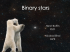### 10.5 The Hertzsprung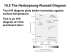### Properties of Stars: The H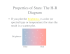### Page 6b Entertainment Page - The Fayetteville Press Newspaper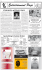### Ancient civilizations already knew that heavenly bodies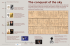### Kepler - STScI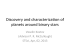### Narrated by Whoopi Goldberg - American Museum of Natural History### Map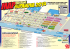### Master Page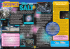### 1230 W. 27TH AVE - Bear Tooth Theatrepub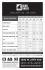### Sunday, February 28, 2016 The 26th Annual "Night of 100 Stars"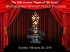### One Sheet - The Kurland Agency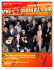### Lyman-α observations of astrospheres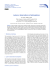### Kornblum, declare - Zeus Legal Notice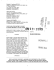### Beta Talasemili Olguda Ekstramedüller Hematopoeze